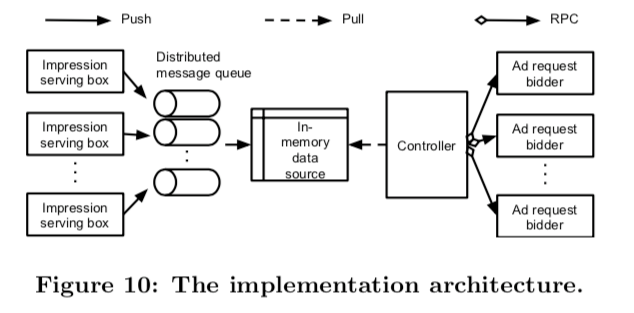Smart Pacing for Effective Online Ad Campaign Optimization》是 Yahoo 在 2015 发表的一篇关于 budget pacing 的论文，与之前写过的 Budget Pacing for Targeted Online Advertisements at LinkedIn 相似，目标也是把预算均匀花完，但是除了这个目标，这篇论文还提出了在预算均匀花完的基础上如何保成本的方法，算是一个多目标优化了。在离线环境和真实环境验证了方法的有效性，是实践性较强的一篇文章，值得一看。

## 背景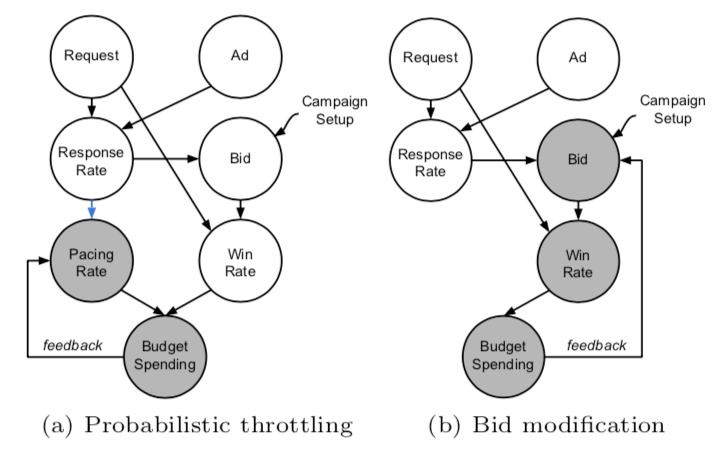1. Probabilistic throttling 是直接影响 budget 花费的速度，而 Bid modification 则是通过改变 win rate 间接达到影响 budget 花费的速度的，这种方式有可能导致 budget 花费波动较大
2. bid landscape(即广告投放中的一些统计信息，能够反映投放的竞争情况，更详细可参考 Bid LandscapesDSP的bidding算法) 一般会随着时间变化而变化；以上两点使得通过 Bid modification 来达到 budget pacing 比较困难
3. 使用 Probabilistic throttling 时能够将 pacing control 与 bid optimization 解耦，能够分别进行优化

## 算法流程

### 问题建模

• $r_i$: 在第 $i$ 个请求参竞的概率，就是本文描述的 pacing rate, $s_i \sim Bern(r_i)$ 则表示是否参竞这个事件
• $w_i$：赢得第 $i$ 个请求的概率
• $c_i$: 赢得第 $i$ 个请求的 cost
• $p_i$: 在第 $i$ 个请求能带来用户点击等行为的概率，$q_i \sim Bern(p_i)$ 则表示是否带来点击这个事件

• $C = \sum_i s_iw_ic_i$ 为计划的总 cost

• $P = \frac{C}{\sum_i s_i w_i q_i}$ 为计划的 performance, 即成本（如点击成本，转化成本等）,文中描述为 eCPC 即点击成本

$$\Omega(B, C) = \sqrt{\frac{1}{K}\sum_{t=1}^{K}(C^{(t)}-B^{(t)})^2}$$

$$\min_{r_i} P \\ s.t\quad C = B, \Omega(C, B) \le \epsilon$$

$$\min_{r_i} \Omega(C, B) \\ s.t\quad P \le G, B-C \le \epsilon$$

### 问题求解

#### Campaigns without Performance Goals

$$\hat{C}^{(t)} = B^{(t)} + \frac{B_m - \sum_{i=t}^KB^{(i)}}{K-m}$$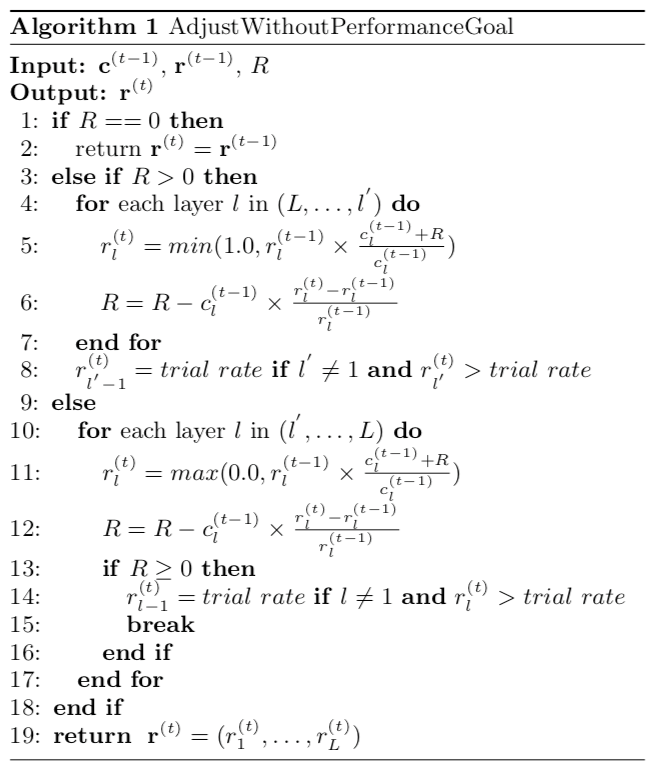1. $l’$ 层表示pacing rate 为非0的最小的层
2. 当需要提升 pacing rate 时，是从第 $L$ 层到 $l’$ 层进行的；而需要降低 pacing rate 时，是从第 $l’$ 层到第 $L$ 层进行的，其目的都是优先提升 ctr 高的层的 pacing rate，优先降低 ctr 低的层的 pacing rate，从而达到上面最小化成本的目的
3. trial rate 的目的令 pacing rate 非 0 的最小层的下一层以一个很小的 pacing rate 进行参竞（pacing rate 会随着层数增加而增加），目的是后面预算花费加速做准备， trail rate在这里是一个超参，是一个很小的值，后面会介绍该如何选取

#### Campaigns with Performance Goals

$$ExpPerf(c^{(t-1)}, r^{(t-1)}, r^{(t)}, e, i) = \frac{\sum_{j=i}^{L} \frac{c_j^{(t-1)} r_j^{(t)}}{r_j^{(t-1)}}}{\sum_{j=i}^{L} \frac{c_j^{(t-1)} r_j^{(t)}}{r_j^{(t-1)}e_j}}$$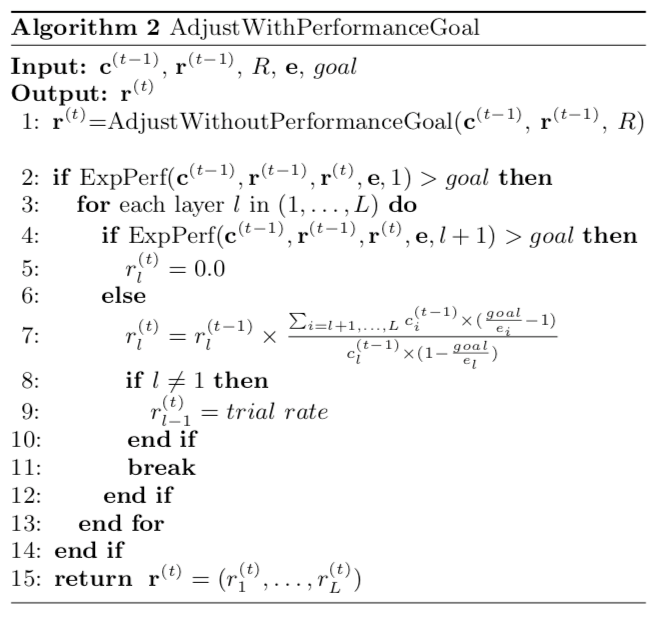1. 如果当前层到第 L 层的联合期望成本大于目标成本，则当前层的 pacing rate 会直接置为 0；
2. 如果当前层到第 L 层的联合期望成本不大于目标成本，当前层的 pacing rate 会根据上面算法第 7 行来调整，然后算法就终止了，根据文章描述其含义是调整当前的层使得总体的期望成本达到目标的期望成本

## 实验效果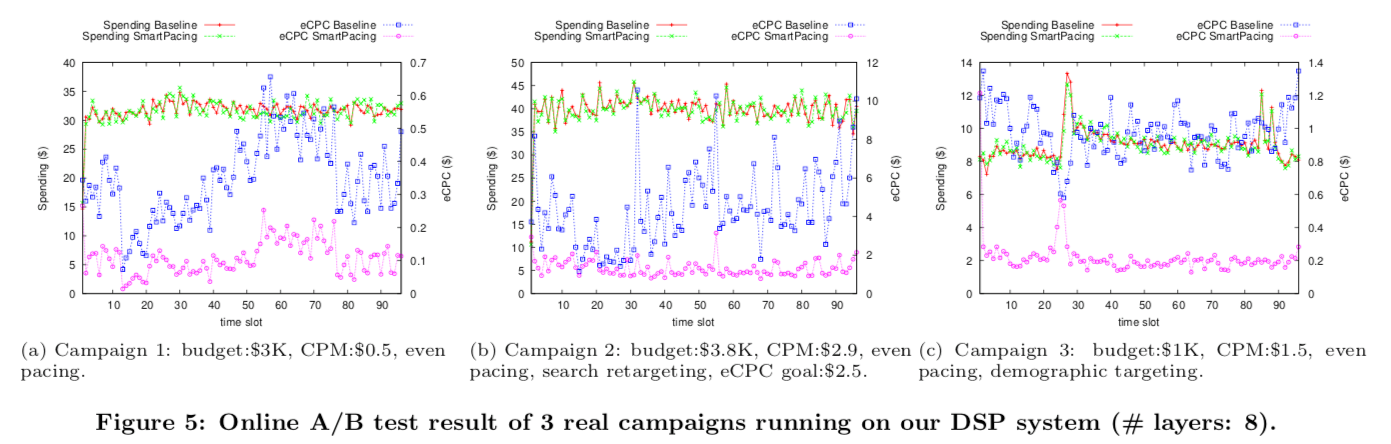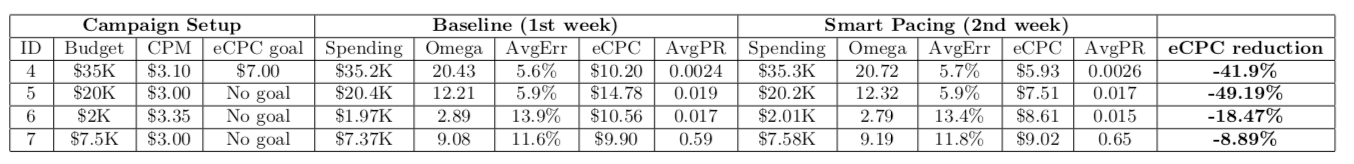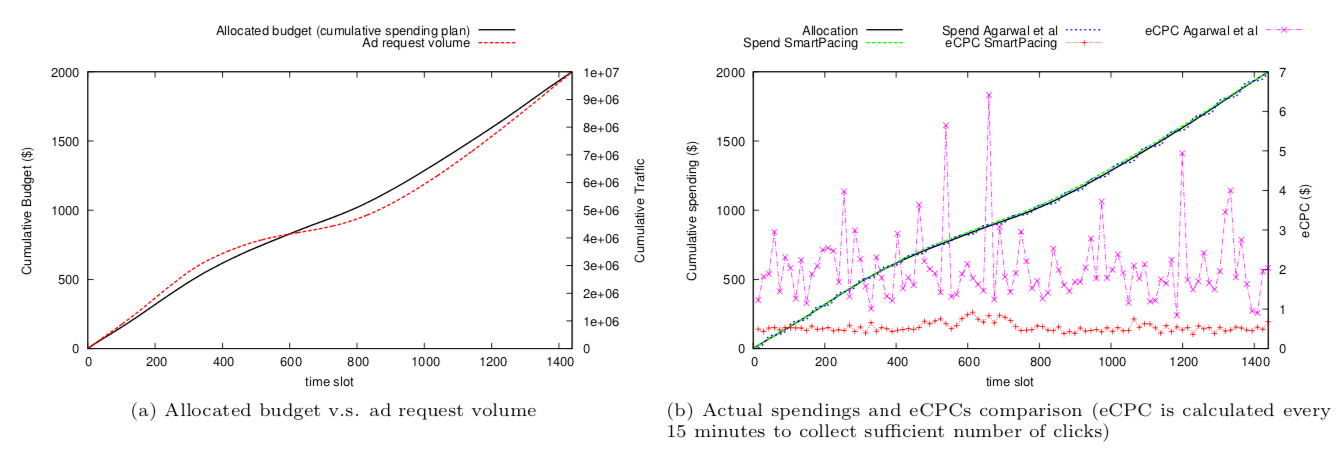## 工程实现